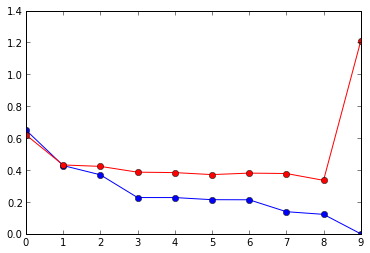In :
%pylab inline

Welcome to pylab, a matplotlib-based Python environment [backend: module://IPython.zmq.pylab.backend_inline].

In :
# Number of samples
N = 10

# Degree of polynomial
M = 4

# Set random seed to make deterministic
random.seed(555)

In :
# The ground truth sinusoid
xground = arange(0, 1, 0.01)
yground = sin(2*pi*xground)

# Get some points at regular interval
x = arange(0, 1, 1./N)
tground = sin(2*pi*x)

plot(xground,yground)
plot(x, tground, 'ro')

Out:
[<matplotlib.lines.Line2D at 0x3a2ddd0>]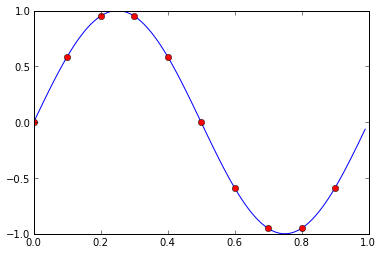In :
# Create our gaussian noise
std_deviation = 0.3
noise = std_deviation * randn(N)
t = tground + noise

plot(xground,yground)
plot(x, t, 'ro')

Out:
[<matplotlib.lines.Line2D at 0x3adef90>]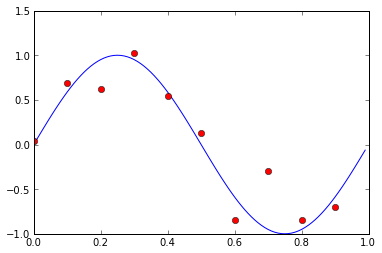In :
# Function to evaluate a polynomial with coefficients w
# (ordered by decreasing degree)
# could also use builtin polyval(w, x)
def polynomial(w, x):
powers = range(0,len(w))
powers.reverse()

powered = array(x)[:,newaxis] ** array(powers)
return dot(powered, w)

# Define our error function
def err(w, x, t):
return 0.5 * sum((polynomial(w,x)-t)**2)

# Root Mean Square error - in same units as data
def rms(w, x, t):
return sqrt(2 * err(w, x, t)/len(x))

In :
# As we shall see, the error can be minimised in closed form
# for the moment, we'll use the built-in function polyfit to do this

w = polyfit(x, t, M)
print w

# Let's see what our polynomial looks like (green)
plot(xground, yground)
plot(x, t, 'ro')
plot(xground, polynomial(w, xground), 'g')

[ -1.78887271e+00   1.98293149e+01  -2.71899014e+01   8.93654375e+00
2.30848663e-02]

Out:
[<matplotlib.lines.Line2D at 0x3d4c490>]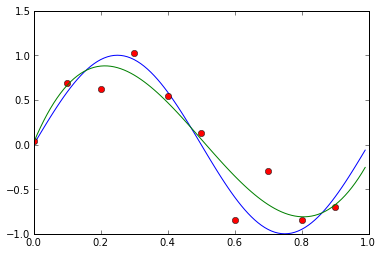In :
# Now we will define a testing set to see
# how well this model works, generated similarly
# to our training data
Ntest = 8
xtest = random_sample(Ntest)
ytest = sin(2*pi*xtest) + randn(Ntest) * std_deviation

plot(xground, polynomial(w, xground), 'g')
plot(x, t, 'ro')
plot(xtest, ytest, 'co')

print "Train Error", err(w, x, t)
print "Test Error", err(w, xtest, ytest)

Train Error 0.258335230389
Test Error 0.589296810631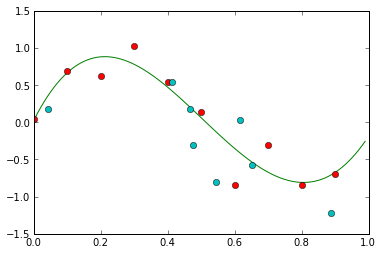In :
# What if we use a higher order polynomial?
w_higher = polyfit(x, t, 9)

plot(x, t, 'ro')
plot(xtest, ytest, 'co')
plot(xground, polyval(w_higher, xground), 'g', scaley=False)

print "Train Error", err(w_higher, x, t)
print "Test Error", err(w_higher, xtest, ytest)

# It passes directly through all our training points, but generalises
# poorly for predicting the test points - overfitting!

Train Error 7.07770892786e-20
Test Error 5.84814955061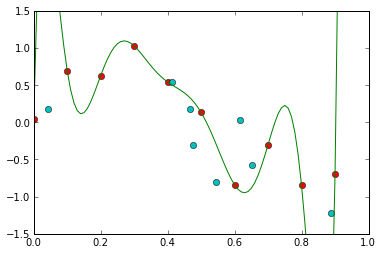In :
# Let's compare the train and test error for different
# orders of polynomial
test_err = []
train_err = []

maxorder = 10

for m in range(0,maxorder):
weights = polyfit(x, t, m)
train_err.append(rms(weights, x, t))
test_err.append(rms(weights, xtest, ytest))

plot(range(0,maxorder), train_err, 'bo-')
plot(range(0,maxorder), test_err, 'ro-')

Out:
[<matplotlib.lines.Line2D at 0x402db10>]